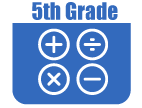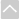OER Curriculum

These Open Educational Resources are comprehensive and coherent curricular materials that may be used to teach a course or grade level.

Engage NY
• Grade 5 Math Module 1: Place Value and Decimal Fractions
In Module 1, students understanding of the patterns in the base ten system are extended from Grade 4s work with place value of multi-digit whole numbers and decimals to hundredths to the thousandths place. In Grade 5, students deepen their knowledge through a more generalized understanding of the relationships between and among adjacent places on the place value chart, e.g., 1 tenth times any digit on the place value chart moves it one place value to the right. Toward the modules end students apply these new understandings as they reason about and perform decimal operations through the hundredths place.
• Grade 5 Math Module 2: Multi-Digit Whole Number and Decimal Fraction Operations
In Module 2 students apply patterns of the base ten system to mental strategies and a sequential study of multiplication via area diagrams and the distributive property leading to fluency with the standard algorithm. Students move from whole numbers to multiplication with decimals, again using place value as a guide to reason and make estimations about products. Multiplication is explored as a method for expressing equivalent measures in both whole number and decimal forms. A similar sequence for division begins concretely with number disks as an introduction to division with multi-digit divisors and leads student to divide multi-digit whole number and decimal dividends by two-digit divisors using a vertical written method. In addition, students evaluate and write expressions, recording their calculations using the associative property and parentheses. Students apply the work of the module to solve multi-step word problems using multi-digit multiplication and division with unknowns representing either the group size or number of groups. An emphasis on the reasonableness of both products and quotients, interpretation of remainders and reasoning about the placement of decimals draws on skills learned throughout the module, including refining knowledge of place value, rounding, and estimation.
• Grade 5 Math Module 3: Addition and Subtraction of Fractions (EngageNY)
In Module 3, students' understanding of addition and subtraction of fractions extends from earlier work with fraction equivalence and decimals. This module marks a significant shift away from the elementary grades' centrality of base ten units to the study and use of the full set of fractional units from Grade 5 forward, especially as applied to algebra.
• Grade 5 Math Module 4: Multiplication and Division of Fractions and Decimal Fractions (EngageNY)
Grade 5's Module 4 extends student understanding of fraction operations to multiplication and division of both fractions and decimal fractions. Work proceeds from interpretation of line plots which include fractional measurements to interpreting fractions as division and reasoning about finding fractions of sets through fraction by whole number multiplication. The module proceeds to fraction by fraction multiplication in both fraction and decimal forms. An understanding of multiplication as scaling and multiplication by n/n as multiplication by 1 allows students to reason about products and convert fractions to decimals and vice versa. Students are introduced to the work of division with fractions and decimal fractions. Division cases are limited to division of whole numbers by unit fractions and unit fractions by whole numbers. Decimal fraction divisors are introduced and equivalent fraction and place value thinking allow student to reason about the size of quotients, calculate quotients and sensibly place decimals in quotients. Throughout the module students are asked to reason about these important concepts by interpreting numerical expressions which include fraction and decimal operations and by persevering in solving real-world, multistep problems which include all fraction operations supported by the use of tape diagrams.
• Grade 5 Math Module 5: Addition and Multiplication with Volume and Area (EngageNY)
In this 25-day module, students work with two- and three-dimensional figures. Volume is introduced to students through concrete exploration of cubic units and culminates with the development of the volume formula for right rectangular prisms. The second half of the module turns to extending students understanding of two-dimensional figures. Students combine prior knowledge of area with newly acquired knowledge of fraction multiplication to determine the area of rectangular figures with fractional side lengths. They then engage in hands-on construction of two-dimensional shapes, developing a foundation for classifying the shapes by reasoning about their attributes. This module fills a gap between Grade 4's work with two-dimensional figures and Grade 6's work with volume and area.
• Grade 5 Math Module 6: Problem Solving with the Coordinate Plane (EngageNY)
In this 40-day module, students develop a coordinate system for the first quadrant of the coordinate plane and use it to solve problems. Students use the familiar number line as an introduction to the idea of a coordinate, and they construct two perpendicular number lines to create a coordinate system on the plane. Students see that just as points on the line can be located by their distance from 0, the planes coordinate system can be used to locate and plot points using two coordinates. They then use the coordinate system to explore relationships between points, ordered pairs, patterns, lines and, more abstractly, the rules that generate them. This study culminates in an exploration of the coordinate plane in real world applications.
•Georgia Standards of Excellence Curriculum Frameworks
• Grade 5 Unit 1: Order of Operations & Whole Numbers (Georgia Standards)
In this unit students will solve problems by representing mathematical relationships between quantities using mathematical expressions and equations. Use the four whole number operations efficiently, including the application of order of operations. Write, evaluate, and interpret mathematical expressions with and without using symbols. Apply strategies for multiplying a 2- or 3-digit number by a 2-digit number. Develop paper-and-pencil multiplication algorithms (the U.S. traditional algorithm is not an expectation) for 3- or 4-digit number multiplied by a 2- or 3-digit number.
• Grade 5 Unit 2: Adding and Subtracting with Decimals (Georgia Standards)
In this unit students will solve problems by understanding that like whole numbers, the location of a digit in a decimal number determines the value of the digit. Understand that rounding decimals should be sensible for the context of the problem. Understand that decimal numbers can be represented with models. Understand that addition and subtraction with decimals are based on the fundamental concept of adding and subtracting the numbers in like position values.
• Grade 5 Unit 3: Multiplying and Dividing with Decimals (Georgia Standards)
General methods used for computing products of whole numbers extend to products of decimals. Because the expectations for decimals are limited to thousandths and expectations for factors are limited to hundredths at this grade level, students will multiply tenths with tenths and tenths with hundredths, but they need not multiply hundredths with hundredths.
• Grade 5 Unit 4: Adding, Subtracting, Multiplying, and Dividing Fractions (Georgia Standards)
Students apply their understanding of fractions and fraction models to represent the addition and subtraction of fractions with unlike denominators as equivalent calculations with like denominators.
• Grade 5 Unit 5: 2D Figures (Georgia Standards)
In this unit students will identify similarities and differences among two-dimensional figures. Reason about attributes (properties) of two-dimensional figures. Have experiences discussing properties of two-dimensional figures. Build upon their fourth-grade knowledge and create a hierarchy diagram. Understand that attributes belonging to a category of two-dimensional figures also belong to all subcategories of that category.
• Grade 5 Unit 6: Volume and Measurement (Georgia Standards)
In this unit students will change units to related units within the same measurement system by multiplying or dividing using conversion factors, use line plots to display a data set of measurements that includes fractions, use operations to solve problems based on data displayed in a line plot and recognize volume as an attribute of three-dimensional space.
• Grade 5 Unit 7: Geometry and the Coordinate Plane (Georgia Standards)
Students should be actively engaged by developing their own understanding. Mathematics should be represented in as many ways as possible by using graphs, tables, pictures, symbols, and words. Appropriate manipulatives and technology should be used to enhance student learning. Students should be given opportunities to revise their work based on teacher feedback, Peer feedback, and metacognition which includes self-assessment and reflection. Students need to write in mathematics class to explain their thinking, talk about how they perceive topics, and justify their work to others.
•The Online Core Resource pages are a collaborative project between the Utah State Board of Education and the Utah Education Network. If you would like to recommend a high quality resource, contact Trish French (Elementary) or Lindsey Henderson (Secondary). If you find inaccuracies or broken links contact resources@uen.org.